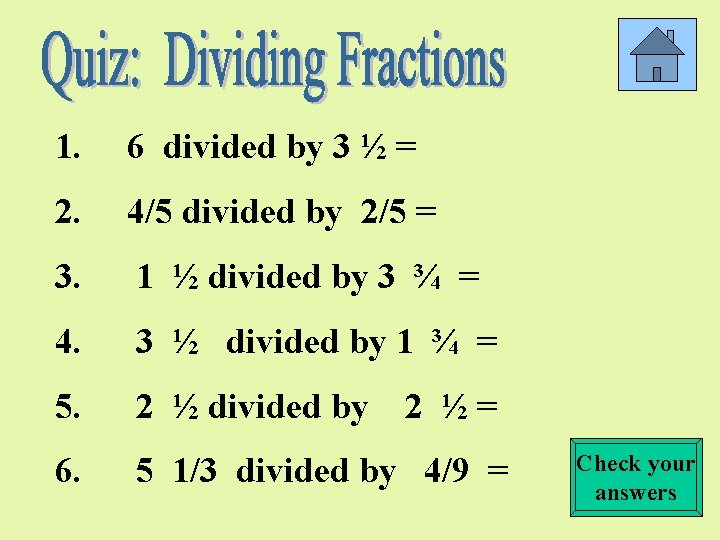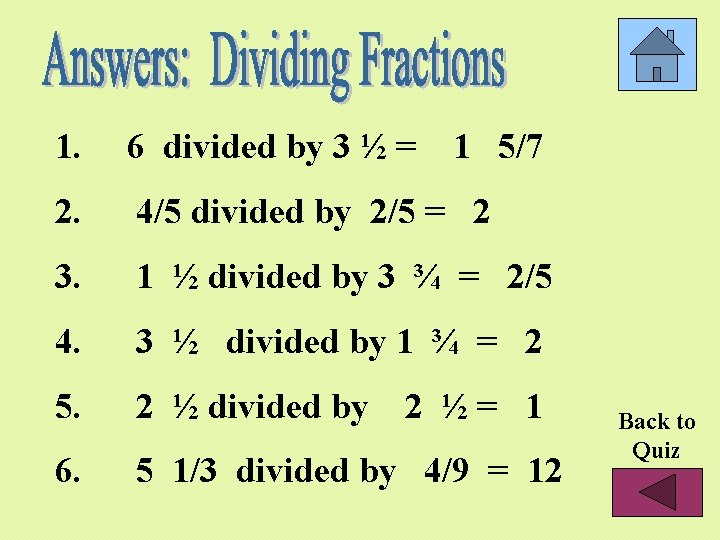# Reciprocal Multiplying Fractions Dividing Fractions QUIZ Multiplying Fractions

• Slides: 12. Reciprocal. Multiplying Fractions. Dividing Fractions QUIZ: Multiplying Fractions QUIZ: Dividing Fractions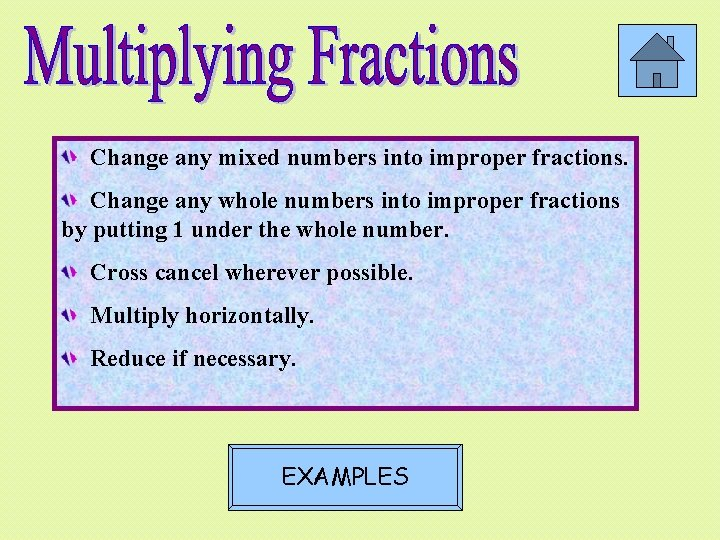Change any mixed numbers into improper fractions. Change any whole numbers into improper fractions by putting 1 under the whole number. Cross cancel wherever possible. Multiply horizontally. Reduce if necessary. EXAMPLES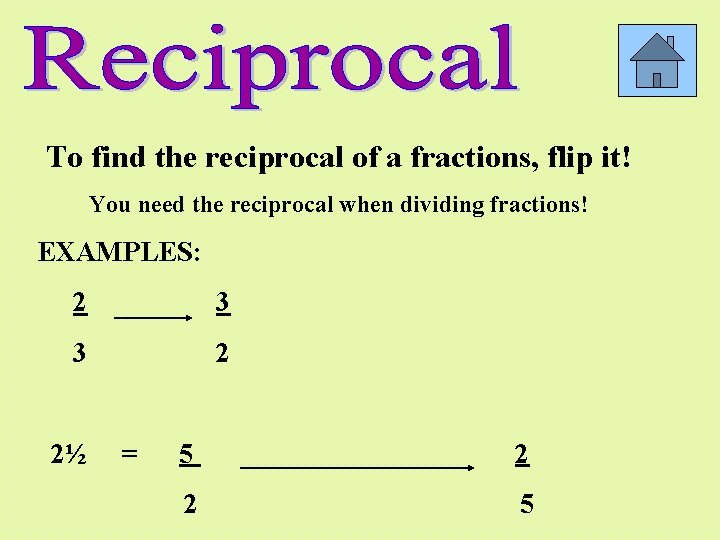To find the reciprocal of a fractions, flip it! You need the reciprocal when dividing fractions! EXAMPLES: 2 3 3 2 2½ = 5 2 2 5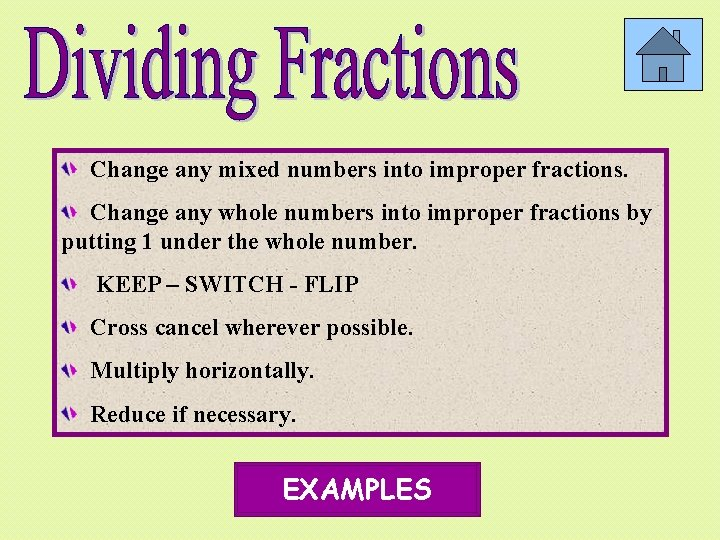Change any mixed numbers into improper fractions. Change any whole numbers into improper fractions by putting 1 under the whole number. KEEP – SWITCH - FLIP Cross cancel wherever possible. Multiply horizontally. Reduce if necessary. EXAMPLESExamples of multiplying fractions 3 8 X 4 1 1 3 9 8 X 4 1 1 Another Example 9 X 2 3 2 1 X 2 = 2 3 1 X 3 = 3Examples of multiplying fractions 2½ X ¼ 5 2 X 1 5 4 8Examples of dividing fractions 5 10 9 12 Another Example FLIP SWITCH KEEP 1 5 3 9 X 12 4 1 10 2 3 x 4 4 2 2 6 3Examples of dividing fractions 3 ½ 2 ¼ 7 9 2 4 FLIP SWITCH KEEP 7 2 x 4 28 9 18 1 10 18 1 5 9